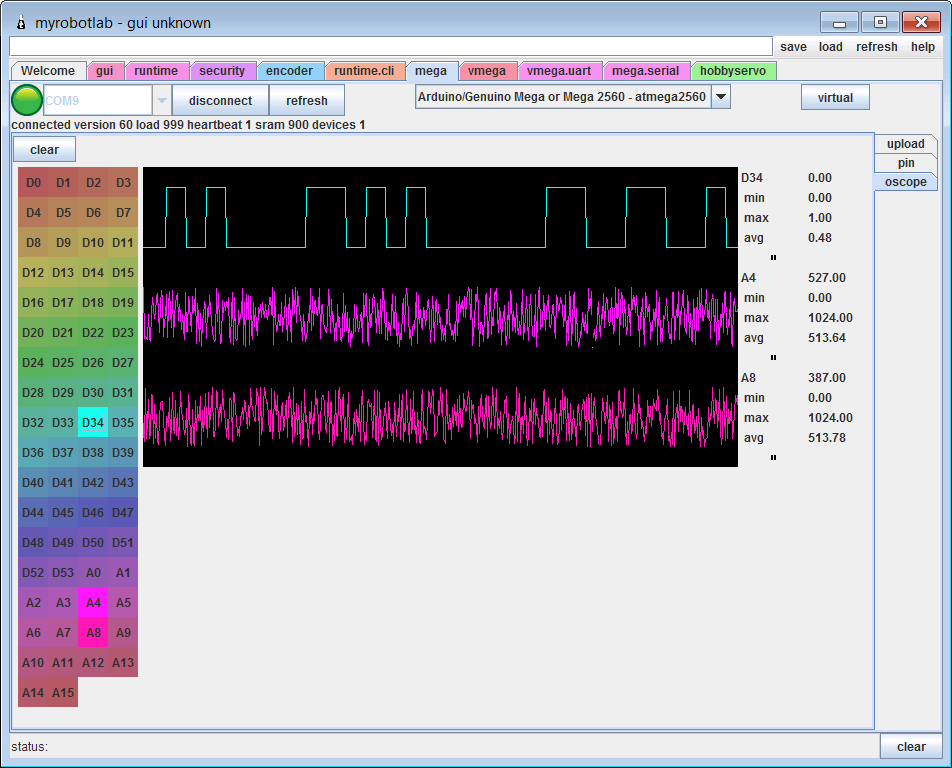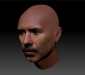# Implementing finger´s sensor

Hi!

We are trying to use the fingers sensor so the robot can grab books of different thickness. Then the idea would be that the fingers close until they detect certain pressure.

I tested the sensors fo the fingers and they work, we can see different values of pressure. But i did this test using an arduino, not using the robot, so i don't know how to add the pressure conditions to the gesture.

Can anyone help us?

Thank you,

Frozen engineers team### Hello and

Hello and Welcome Caroline-EXmachina !

(really like the name)

I assume your doing this in Python.
You have an analog sensor reading from one of the analog pins, and you want to feed that information back into the positioning of the servo ?

Is that correct ?

Have you used myrobotlab before ? or only Arduino sketches ?

myrobotlab comes with a oscope, where the pressure can be visualized.
This is an example of the latest with 2 analog pins and 1 digital reading samplesWith myrobotlab you can write a "callback" function in python which will deliver the pressure data to a function which you can use to determine position of servo & finger.

Is this what you are trying to do ?### Hello, Here is a example

Hello,
Here is a example python script you can use to test the sensor into MyRobotLab.
This script will work with Manticore version. Just execute it in the python tab after launching myrobotlab.jar
The COM port has to be modified to your Arduino com port.
The sensor has to be connected to pin A0.
In this script I was testing a hall sensor which doesn't give the same values as the foam sensor, therefore you will need to modify those input values.
#This simple sensor InMoov script is tested on MyRobotLab version 1.0.2693
#The result can be seen in the Oscope and with the finger action.

leftPort = "COM10"

Voice="0"
mouth = Runtime.createAndStart("i01.mouth", "LocalSpeech")
mouth.setVoice(Voice)
#mouth.setRate(-10)

i01 = Runtime.createAndStart("i01", "InMoov")
i01.startMouth()
leftHand = Runtime.create("i01.leftHand","InMoovHand")
i01.startLeftHand(leftPort)
i01.leftHand.index.map(0,180,25,165)

left=Runtime.create("i01.left", "Arduino")
left.setBoard("atmega2560")
left = Runtime.start("i01.left", "Arduino")
left.connect("COM10")

def publishPin(pins):
for pin in range(0, len(pins)):
if pins[pin].value<=540:
print "No pressure"
if pins[pin].value>=541 and pins[pin].value<=542:
print "Low pressure"
if pins[pin].value>=543 and pins[pin].value<=544:
print "Soft pressure"
FingerGoesBack()
if pins[pin].value>=545:
print "High pressure"
FingerGoesBack()
i01.mouth.speak(u"OUCH, that hurts")

sleep(5)
left.enablePin(54,1) # 54 is pin A0, Number 1 is how many polls/second

i01.leftHand.setAutoDisable(True)

def moveFingerSlowly():
i01.leftHand.index.enable()
i01.leftHand.index.setVelocity(25)
sleep(2)
i01.leftHand.index.moveTo(180)

def FingerGoesBack():
i01.leftHand.index.enable()
i01.leftHand.index.setVelocity(-1)
i01.leftHand.index.moveTo(0)